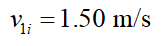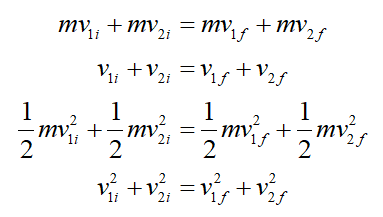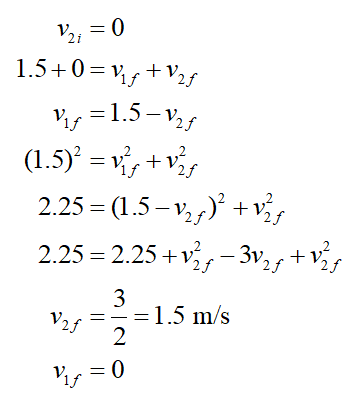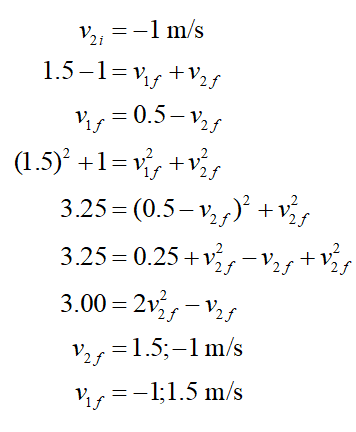# A billiard ball rolling across a table at 1.50 m/s makes a head-on elastic collision with an identical ball. Find the speed of each ball after the collision (a) when the second ball is initially at rest, (b) when the second ball is moving toward the first at a speed of 1.00 m/s, and (c) when the second ball is moving away from the first at a speed of 1.00 m/s.

Question
35 views

A billiard ball rolling across a table at 1.50 m/s makes a head-on elastic collision with an identical ball. Find the speed of each ball after the collision (a) when the second ball is initially at rest, (b) when the second ball is moving toward the first at a speed of 1.00 m/s, and (c) when the second ball is moving away from the first at a speed of 1.00 m/s.

check_circle

Step 1

Let m be the mass of the billiard ball,Using conservation of energy and momentum we will find the unknowns,Step 2

a) It is given that second ball is initially at rest,Step 3

b) The second ball is moving towards first ball with speed of 1 m/s....

### Want to see the full answer?

See Solution

#### Want to see this answer and more?

Solutions are written by subject experts who are available 24/7. Questions are typically answered within 1 hour.*

See Solution
*Response times may vary by subject and question.
Tagged in